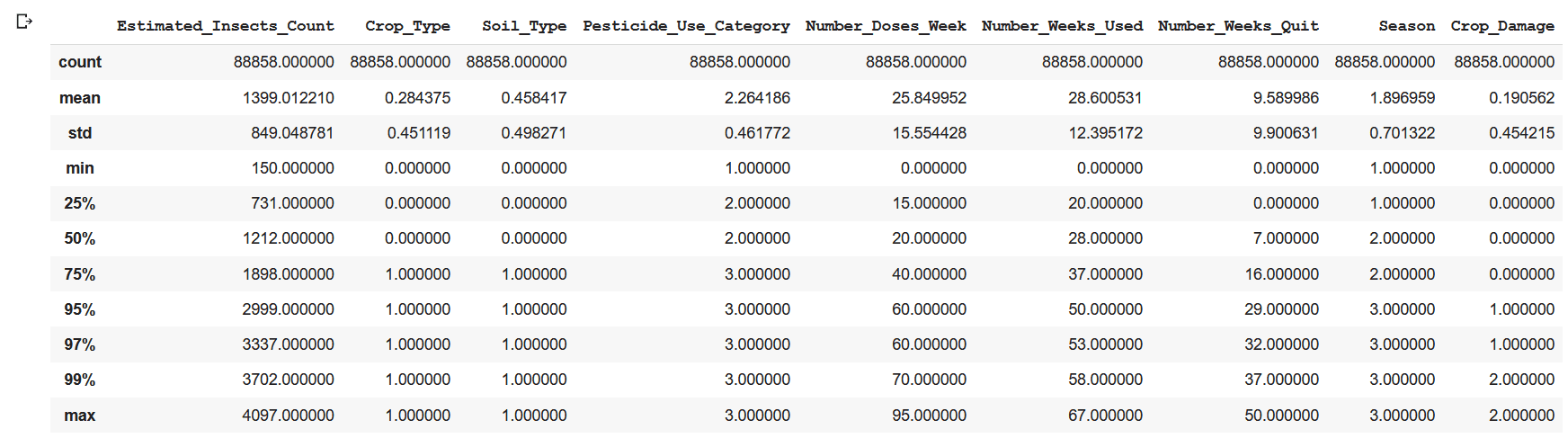# EDA : THE HIDDEN SECRET OF DATAROSHAN KUMAR G Oct 14 2020 · 4 min read

### Why exploratory data analysis (EDA) ?

Exploratory data analysis is an approach to analyze the data. It's where a data enthusiast would be able to get an idea of overall structure of a dataset by bird's eye view. Data science often consist of advanced statistical and machine learning techniques. However, often the power of exploratory data analysis (EDA) is underestimated. In statistics, exploratory data analysis is an approach to analyzing dataset to summarize their main characteristics, often with visual methods. EDA is capable of telling us what kind of statistical techniques or modelling can be applied for the data.

EDA also plays a important role in feature engineering part as well. Having a good idea about the features in the data set, we will be able to create more significant features.

### Main purpose of EDA

• Check the missing values in data or any irrelevant characters
• Detect the Anomalies/Outliers in data
• Understand each and every data point by various analysis techniques
• Analyze the relationship between the variables
• ### General steps followed

• Missing value treatment
• Univariate Analysis
• Bivariate Analysis
• Multivariate Analysis
• Outlier treatment
• Correlation Analysis
• Dimensionality Reduction

Library Imports

``import pandas as pd import numpy as npimport matplotlib.pyplot as pltimport seaborn as sns import warningswarnings.filterwarnings('ignore')``

Importing Dataset:

``data = pd.read_csv("train_yaOffsB(1).csv")data.shape ``

Here we will be able to observe that dataset has (88858, 10) , 10 features and 88858 data points.

``data.head(3).append(data.tail(3))data['ID'].nunique() ``

Missing value analysis

``import missingno as msnoprint(data.isnull().sum())p = msno.bar(data, figsize = (9,6))``
``data.info()``From the info() function we can get the data types of the variables along with the non-null values present in each column
``data['Number_Weeks_Used'].fillna(method = 'ffill', inplace = True)data['Number_Weeks_Used'] = data['Number_Weeks_Used'].astype('int64')``

Here i have used forward fill to impute the missing values just for simplicity, you could use any of the methods such as mean, median , mode etc..or just drop the missing values.

Summary of Data

``col = data.columns.tolist()col.remove('ID')data[col].describe(percentiles = [.25,.5,.75,.95,.97,.99])  ``Pandas describe() function provides the statistical summary about the data such as mean, max, min, standard deviation, count along with this we can also pass the percentiles where we will be able to get the idea about the outliers in the data.

Filtering data based on condition

``data[(data['Season'] == 1) & (data['Crop_Damage'] == 1) & (data['Soil_Type'] == 0)].head() pd.DataFrame(data.groupby(['Crop_Damage','Crop_Type'])['Pesticide_Use_Category'].count())pd.DataFrame(data.groupby(['Crop_Damage','Season','Crop_Type'])['Estimated_Insects_Count'].count())``
``df = pd.DataFrame( data[data['Crop_Damage'] == 1 ].mean(), columns = ['Values'])df[ 'Variance'] = pd.DataFrame( data[data['Crop_Damage'] == 1 ].var())df[ 'Standard deviation'] = pd.DataFrame( data[data['Crop_Damage'] == 1 ].std())df[ 'Median'] = pd.DataFrame( data[data['Crop_Damage'] == 1 ].median())df``

Graphical analysis

``plt.subplot(1,2,1)sns.countplot(x = 'Crop_Damage' , palette= 'cool', data= data) plt.title("Count plot of Crop damage (target variable)")plt.subplot(1,2,2)count = train['Crop_Damage'].value_counts()count.plot.pie(autopct = '%1.1f%%',colors=['green','orange','blue'], figsize = (10,7),explode = [0,0.1,0.1],title = "Pie chart of Percentage of Crop_Damage")``

By the count plot and pie chart we can infer that crop alive category has larger data points as compared to the other two categories. Since this is a multi-class classification problem, this is a clear case of multi-class imbalance problem.

``plt.figure(figsize = (10,6))plt.subplot(1,2,1)sns.countplot(x = 'Crop_Type' , palette= 'cool', data= data) plt.title("Count plot of Crop_Type")plt.subplot(1,2,2)sns.countplot(data['Crop_Type'], hue = data['Crop_Damage'],palette="rocket_r")plt.title("Plot of crop damage Vs Crop type")``

Inference

* Crop type 0 has larger data points as compared to the crop type 1

* More than 50000 of the crops of crop type 0 and 20000 of crops of crop type 1 are alive

* There is more damage to crop 0 due to pesticides

``plt.figure(figsize = (15,5))sns.countplot(data['Number_Weeks_Used'], palette = 'hsv')plt.title('Count of Number_Weeks_Used')plt.show() sns.countplot(data['Number_Doses_Week'], palette = 'hsv')plt.title('Count of Number_Doses_Week')plt.show() ``

Inference

*By the above plot we can conclude that week 20 and week 30 has larger proportion

* In the number of doses per week we observe that dose 20 has greater proportion

``sns.distplot(data['Estimated_Insects_Count'], kde = True, hist = True, rug= False, bins= 30)plt.title("Density plot of Estimated_Insects_Count")``
``plt.figure(figsize = (10,5))plt.subplot(1,2,1)sns.countplot(data['Season'], palette = 'hsv')plt.title('Count plot of Season')plt.subplot(1,2,2)sns.countplot(data['Season'], hue = data['Crop_Damage'], palette = 'hsv')plt.title('Count plot of Crop_Damage in Seasons')plt.show() ``

Inference

* From the density plot we observe that Estimated insects count is right skewed

* Count plot of crop damage at different seasons provides us the idea that, the crop damage is more  in season 1

``sns.countplot(data['Season'], hue = data['Crop_Type'])plt.title('Count plot of Crop_type in Seasons')``
``sns.countplot(data['Pesticide_Use_Category'], palette = 'dark')plt.title("Count plot of Pesticide_Use_Category")plt.show()sns.catplot(x = 'Pesticide_Use_Category', y = 'Estimated_Insects_Count', kind = 'box', data = data, hue = 'Crop_Damage', palette= 'rocket_r')plt.title("Box plot of Pesticide_Use_Category")``

Information included in Box plot

* Minimum

* First Quartile

* Median (Second Quartile)

* Third Quartile

* Maximum

* Idea about outliers in data

``data[col].hist(figsize=(10,15),color = 'green')``